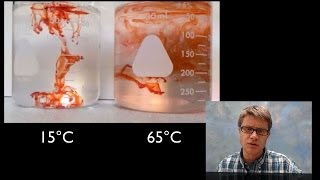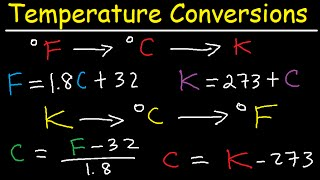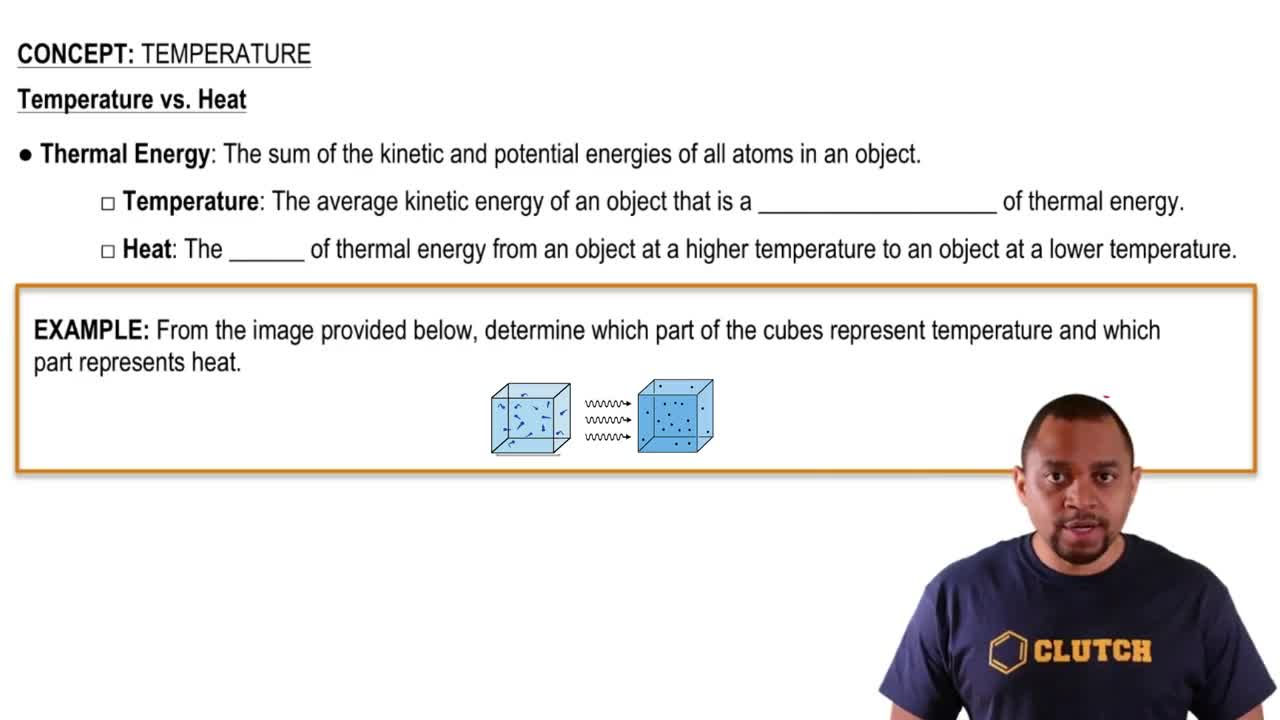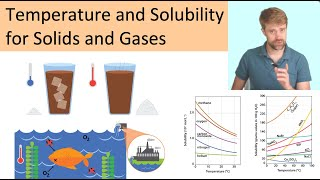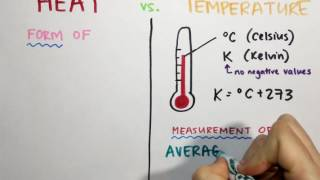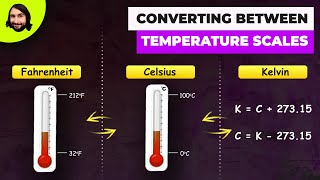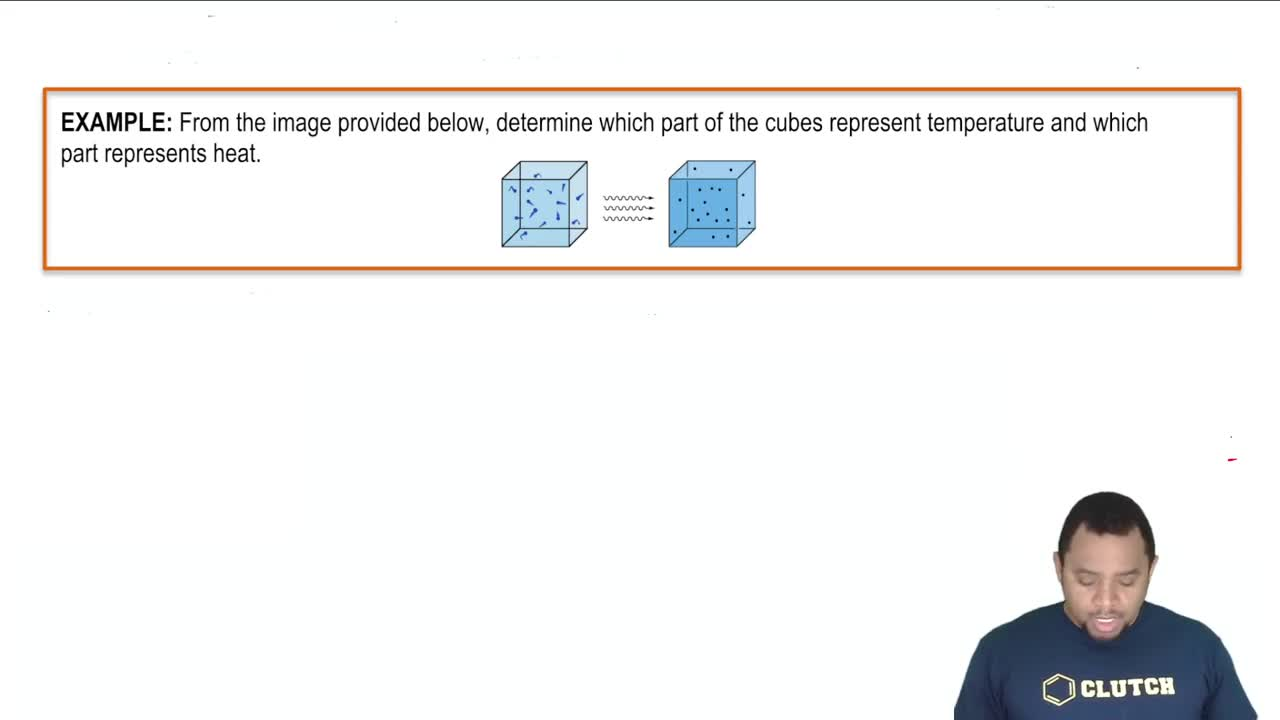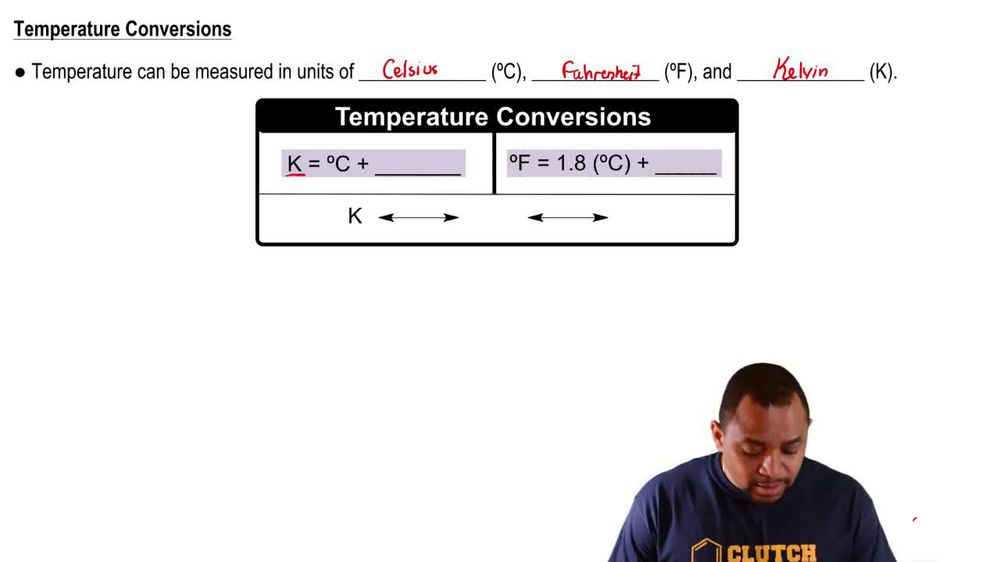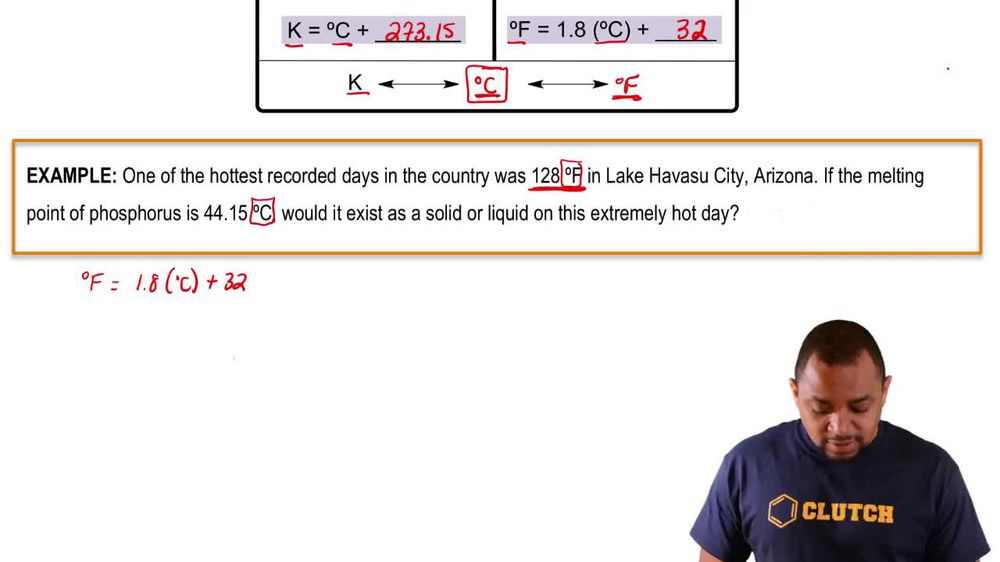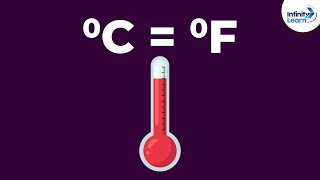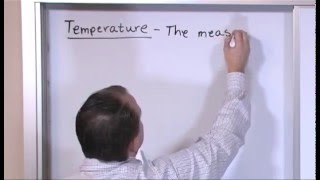Start typing, then use the up and down arrows to select an option from the list.
1. 1. Intro to General Chemistry2. Temperature
Problem

# Suppose you were dissatisfied with both Celsius and Fahrenheit units and wanted to design your own temperature scale based on ethyl alcohol (ethanol). On the Celsius scale, ethanol has a melting point of -117.3 °C and a boiling point of 78.5 °C, but on your new scale calibrated in units of degrees ethanol, °E, you define ethanol to melt at 0 °E and boil at 200 °E. (b) How does an ethanol degree compare in size with a Fahrenheit degree?

Relevant Solution4m
Play a video:
Hi everyone, this problem reads suppose you wanted to create your own temperature scale based on propose alcohol since you are unsatisfied with both the Celsius and Fahrenheit units the melting point and boiling point of propane all on the Celsius scale are negative 126 degrees Celsius and 97.2 degrees Celsius, respectively. In the new scale, you define the melting point and boiling point of propane all at zero degrees P and and 50 degrees P respectively in terms of size, how does an ethanol degree compare to a Fahrenheit degree? So this is the question we want to answer. How does an ethanol degree compare to a Fahrenheit degree? So here we know what our temperature is in degrees Celsius for both the boiling point and melting point. So let's go ahead and start there. We're going to write out propane all boiling point and degrees Celsius is 97.2 degrees Celsius and propane all's melting point and degrees Celsius is negative 126 degrees Celsius. The question is asking us to compare the ethanol degree which is degrees P to the Fahrenheit degree. So we need to convert our degrees Celsius to Fahrenheit. Okay, so let's go ahead and do that for the boiling point. We're going to take to figure out its degrees in Fahrenheit, we're going to take its degrees Celsius. So 97.2 degrees Celsius. We're going to multiply this by 9/5 plus 32 is going to give us 206. degrees Fahrenheit. Okay, so same thing for melting point. We need to convert it to Fahrenheit. So we're going to take its temperature and Celsius. So negative 126 degrees Celsius times 9/ plus 32 gives us a temperature of negative 94.8 degrees Fahrenheit. So let's go ahead and write those next to our degrees Celsius. So 206.96 degrees Fahrenheit and negative 194 0. degrees Fahrenheit. And we know our new scale. The temperature for a new scale for the boiling point we're told in the problem is 100 and 50 P. And the temperature for a melting point on the ethanol scale is zero degrees P. So we want to know or calculate the size difference in terms of size. How does ethanol degree compare to Fahrenheit degree. So in order for us to calculate the size difference, what we're going to do is we're going to take the boiling point in degrees P minus the melting point and degrees P. And we're going to divide that by the boiling point and degrees Fahrenheit minus the melting point and degrees Fahrenheit. Okay, so let's go ahead and plug those values in. So we have for a boiling point in degrees p boiling point and degrees P we have 100 and minus melting point and degrees p is zero over our boiling point in degrees Fahrenheit. So this is 206. minus our melting point and degrees Fahrenheit so negative. 1 94.8. Okay, so once we get this calculated, our size difference equals 0. 3357 degrees P over degrees Fahrenheit. Okay, so this is going to be our size difference and that is the answer to this problem. I hope this was helpful.